General Linear Model

# General Linear Model

The General Linear Model (GLM) underlies most of the statistical analyses that are used in applied and social research. It is the foundation for the t-test, Analysis of Variance (ANOVA), Analysis of Covariance (ANCOVA), regression analysis, and many of the multivariate methods including factor analysis, cluster analysis, multidimensional scaling, discriminant function analysis, canonical correlation, and others. Because of its generality, the model is important for students of social research. Although a deep understanding of the GLM requires some advanced statistics training, I will attempt here to introduce the concept and provide a non-statistical description.

## The Two-Variable Linear Model

The easiest point of entry into understanding the GLM is with the two-variable case. Figure 1 shows a bivariate plot of two variables. These may be any two continuous variables but, in the discussion that follows we will think of them as a pretest (on the x-axis) and a posttest (on the y-axis). Each dot on the plot represents the pretest and posttest score for an individual. The pattern clearly shows a positive relationship because, in general, people with higher pretest scores also have higher posttests, and vice versa.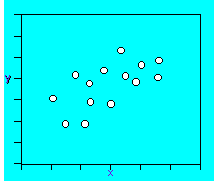Figure 1. Bivariate plot.

The goal in our data analysis is to summarize or describe accurately what is happening in the data. The bivariate plot shows the data. How might we best summarize these data? Figure 2 shows that a straight line through the “cloud” of data points would effectively describe the pattern in the bivariate plot. Although the line does not perfectly describe any specific point (because no point falls precisely on the line), it does accurately describe the pattern in the data. When we fit a line to data, we are using what we call a linear model. The term “linear” refers to the fact that we are fitting a line. The term model refers to the equation that summarizes the line that we fit. A line like the one shown in Figure 2 is often referred to as a regression line and the analysis that produces it is often called regression analysis.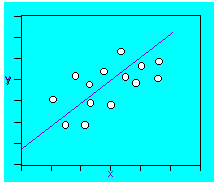Figure 2. A straight-line summary of the data.

Figure 3 shows the equation for a straight line. You may remember this equation from your high school algebra classes where it is often stated in the form:

$$y = mx + b$$

In this equation, the components are:

• y = the y-axis variable, the outcome or posttest
• x = the x-axis variable, the pretest
• b0 = the intercept (value of y when x=0)
• b1 = the slope of the line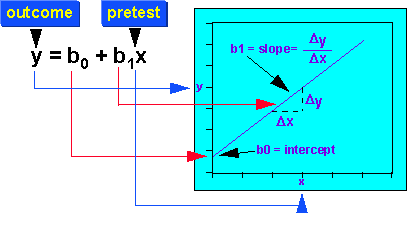Figure 3. The straight-line model.

The slope of the line is the change in the posttest given in pretest units. As mentioned above, this equation does not perfectly fit the cloud of points in Figure 1. If it did, every point would fall on the line. We need one more component to describe the way this line is fit to the bivariate plot.

Figure 4 shows the equation for the two variable or bivariate linear model. The component that we have added to the equation in Figure 3 is an error term, e, that describes the vertical distance from the straight line to each point. This term is called “error” because it is the degree to which the line is in error in describing each point.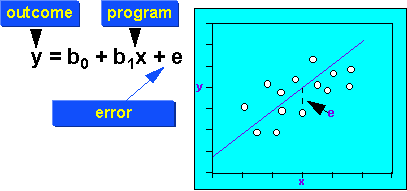Figure 4. The two-variable linear model.

When we fit the two-variable linear model to our data, we have an x and y score for each person in our study. We input these value pairs into a computer program. The program estimates the b0 and b1 values for us as indicated in Figure 5. We will actually get two numbers back that are estimates of those two values.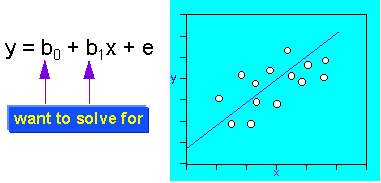Figure 5. What the model estimates.

You can think of the two-variable regression line like any other descriptive statistic – it is simply describing the relationship between two variables much as a mean describes the central tendency of a single variable. And, just as the mean does not accurately represent every value in a distribution, the regression line does not accurately represent every value in the bivariate distribution. We use these summaries because they show the general patterns in our data and allow us to describe these patterns in more concise ways than showing the entire distribution allows.

## The General Linear Model

Given this brief introduction to the two-variable case, we are able to extend the model to its most general case. Essentially the GLM looks the same as the two variable model shown in Figure 4 – it is just an equation. But the big difference is that each of the four terms in the GLM can represent a set of variables, not just a single one. So, the general linear model can be written:

$$y=b_0+b_x+e$$

where:

• y = a set of outcome variables

• x = a set of pre-program variables or covariates

• b0 = the set of intercepts (value of each y when each x = 0)

• b = a set of coefficients, one each for each x

You should be able to see that this model allows us to include an enormous amount of information. In an experimental or quasi-experimental study, we would represent the program or treatment with one or more dummy coded variables, each represented in the equation as an additional x-value (although we usually use the symbol z to indicate that the variable is a dummy-coded x). If our study has multiple outcome variables, we can include them as a set of y-values. If we have multiple pretests, we can include them as a set of x-values. For each x-value (and each z-value) we estimate a b-value that represents an x,y relationship. The estimates of these b-values, and the statistical testing of these estimates, is what enables us to test specific research hypotheses about relationships between variables or differences between groups.

The GLM allows us to summarize a wide variety of research outcomes. The major problem for the researcher who uses the GLM is model specification. The researcher is responsible for specifying the exact equation that best summarizes the data for a study. If the model is misspecified, the estimates of the coefficients (the b-values) are likely to be biased (i.e., wrong) and the resulting equation will not describe the data accurately. In complex situations, this model specification problem can be a serious and difficult one (see, for example, the discussion of model specification in the statistical analysis of the regression-discontinuity design).

The GLM is one of the most important tools in the statistical analysis of data. It represents a major achievement in the advancement of social research in the twentieth century.